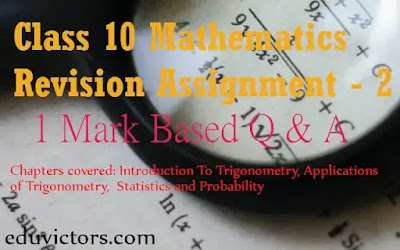# Class 10 Maths Revision Assignment -2

1-Mark Questions

Chapters: Introduction to Trigonometry, Applications of Trigonometry, Statistics, Probability

Click on the image to see the zoomed view.
1: 60°
2: A= 45°, B = 15°
3: elevation is 60°
4: 21.7m
5: 17, 62.5
6: modal class = 30 -40
7: False, since outcomes of Head and Tail are equally likely
8: P(E) = 1/8

CH 1: Real Numbers (Important Formulae and Key Points)

CH 9: Applications of Trigonometry (MCQs)

CH 15: 5 Minutes Revision on Probability

Maths Revision Assignment -1  (1-Mark Questions) (Solved)